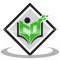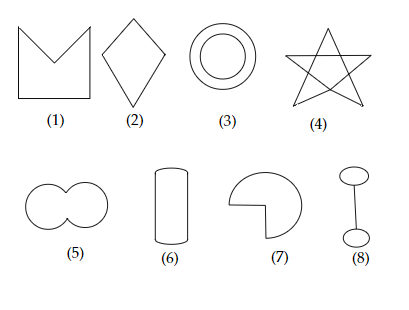## What is a regular polygon?State the name of a regular polygon of(i) 3 sides(ii) 4 sides(iii) 6 sides.Updated on 10-Oct-2022 13:47:56
To do:  We have to define regular polygon and state the name of a regular polygon of(i) 3 sides(ii) 4 sides(iii) 6 sides.Solution:A polygon is a closed plane or two-dimensional figure whose sides are straight line segments.Regular polygon:A regular polygon is a polygon that has equal sides and equal angles.(i) A regular polygon of 3 sides is called an equilateral triangle.(ii) A regular polygon of 4 sides is called a square.(iii) A regular polygon of 6 sides is called regular hexagon.

## How many diagonals does each of the following have?(a) A convex quadrilateral(b) A regular hexagon(c) A triangle.Updated on 10-Oct-2022 13:47:54
To do:  We have to find the number of diagonals each of the below shapes have.(a) A convex quadrilateral(b) A regular hexagon(c) A triangle.Solution:(a) A convex quadrilateral is shown below.From the above figure, we can observe that,A convex quadrilateral has two diagonals.(b) A regular hexagon is shown below.From the above figure, we can observe that,A regular hexagon has nine diagonals.(c) A triangle is shown below.From the above figure, we can observe that,A triangle has no diagonals.

## Given here are some figures.Classify each of them on the basis of the following.(a) Simple curve(b) Simple closed curve(c) Polygon(d) Convex polygon(e) Concave polygon."Updated on 10-Oct-2022 13:47:53
To do:  We have to classify each of the given figures on the basis of the following.(a) Simple curve(b) Simple closed curve(c) Polygon(d) Convex polygon(e) Concave polygon.Solution:We know that, Simple curves:Simple curves do not cross or intersect.Simple closed curve:Does not have end points and does not intersect.Polygon:A polygon is a closed plane or two-dimensional figure whose sides are straight line segments. Convex Polygon: A polygon is convex if it contains all the line segments connecting any pair of its points.Concave Polygon: A polygon that is not convex is said to be a concave polygon.Therefore, (a) Figures 1, 2, 5, 6 and 7 are simple ... Read More

## If $(2x-1,3y+1)$ and $(x+3,y-4)$ are equal ordered pairs. Find the values of $x$ and $y$.Updated on 10-Oct-2022 13:47:41

## In one fortnight of a given month, there was a rainfall of $10 \mathrm{~cm}$ in a river valley. If the area of the valley is $7280 \mathrm{~km}^{2}$, show that the total rainfall was approximately equivalent to the addition to the normal water of three rivers each $1072 \mathrm{~km}$ long, $75 \mathrm{~m}$ wide and $3 \mathrm{~m}$ deep.Updated on 10-Oct-2022 13:47:38
Given:In one fortnight of a given month, there was a rainfall of $10 \mathrm{~cm}$ in a river valley. The area of the valley is $7280 \mathrm{~km}^{2}$.To do:We have to show that the total rainfall was approximately equivalent to the addition to the normal water of three rivers each $1072 \mathrm{~km}$ long, $75 \mathrm{~m}$ wide and $3 \mathrm{~m}$ deep.Solution:Height of the rain $=10\ cm$$=\frac{10}{100}\ m$$=\frac{10}{100\times1000}\ km$Total rainfall in the river valley $=$ area of the valley $\times$ height of the rain$=7280\times\frac{10}{100\times1000}$$=0.7280\ km^3Volume of normal water in each river =1072\times\frac{75}{1000}\times\frac{3}{1000}$$= 0.2412\ km^3$Volume ... Read More

## A cistern, internally measuring $150 \mathrm{~cm} \times 120 \mathrm{~cm} \times 110 \mathrm{~cm}$, has $129600 \mathrm{~cm}^{3}$ of water in it. Porous bricks are placed in the water until the cistern is full to the brim. Each brick absorbs one-seventeenth of its own volume of water. How many bricks can be put in without overflowing the water, each brick being $22.5 \mathrm{~cm} \times 7.5 \mathrm{~cm} \times 6.5 \mathrm{~cm}$ ?Updated on 10-Oct-2022 13:47:38